The LIFEREG Procedure
 Confidence Intervals

Confidence intervals are computed for all model parameters and are reported in the "Analysis of Parameter Estimates" table. The confidence coefficient can be specified with the ALPHA=MODEL statement option, resulting in a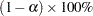two-sided confidence coefficient. The default confidence coefficient is 95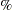, corresponding to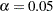.

### Regression Parameters

A two-sidedconfidence interval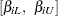for the regression parameter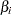is based on the asymptotic normality of the maximum likelihood estimator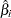and is computed by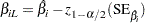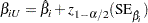where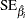is the estimated standard error of, and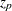is the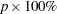percentile of the standard normal distribution.

### Scale Parameter

A two-sidedconfidence interval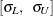for the scale parameter
in the location-scale model is based on the asymptotic normality of the logarithm of the maximum likelihood estimator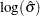, and is computed by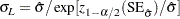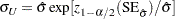Refer to Meeker and Escobar (1998) for more information.

### Weibull Scale and Shape Parameters

The Weibull distribution scale parameter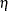and shape parameter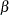are obtained by transforming the extreme-value location parameterand scale parameter: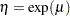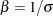Consequently, two-sidedconfidence intervals for the Weibull scale and shape parameters are computed as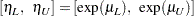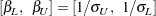### Gamma Shape Parameter

A two-sidedconfidence interval for the three-parameter gamma shape parameter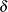is computed by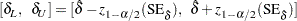Previous Page | Next Page | Top of Page